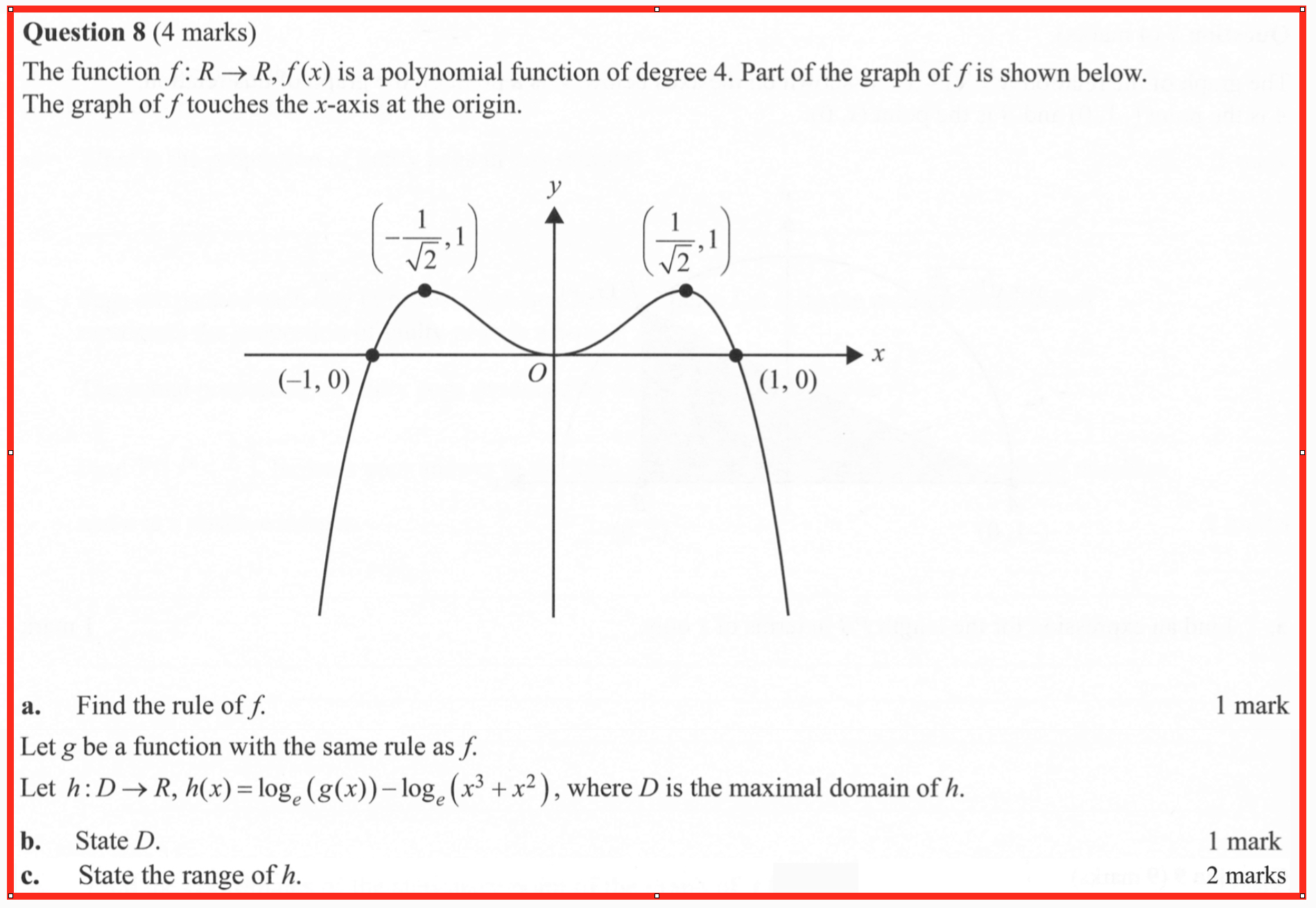# WitCH 27: Uncomposed

Ah, so much crap …

Tons of nonsense to post on, and the Evil Mathologer is breathing down our neck. We’ll have (at least) three posts on last week’s Mathematical Methods exams. This one is by no means the worst to come, but it fits in with our previous WitCH, so let’s quickly get it going. It is from Exam 1. (No link yet, but the Study Design is here.)## 8 Replies to “WitCH 27: Uncomposed”

1.Glen Wheeler says:

“Find the rule of f”. Why does this annoy me so much?

I suppose it’s because I’d much rather them just ask for the roots with multiplicity.

What is the point of g? Am I missing something with this “rule” idea? Are f and g not the same thing?

What is the point of subtracting the second logarithm? The method to solve the problem is the same.

Is “maximal domain” ever actually defined for these students? I really doubt it.

Why is the last part worth 2 marks? If they just want a statement, it is going to be all or nothing.

Argh these questions are infuriating!

2.Red Five says:

Seconded. Does “the rule” include the domain or not??? (picture me yelling at a cloud similar to Abe Simpson).

Because the wording of part b implies “the rule” does not include the domain. In which case why didn’t they say “the equation”??? (keeps yelling at cloud)

And the 2 marks for the final part is usually (not always) a clue that there are two parts to the answer…

1.Glen Wheeler says:

Right, that’s why I’m confused with the two marks. Because how could you mark it wrong if a student just wrote “(-\infty, \log 4]”? (Assuming my working is right.)

1.Glen Wheeler says:

(-\infty, \log 8] I guess? Bah.

3.amca01 says:

Sloppy in the extreme. The question already says that f is defined by a fourth degree polynomial. So why not, in (a), simply ask for the polynomial? And what do they mean by g “having the same rule as f”? This makes no sense at all. If what they mean is that f will from now on have a restricted domain, that’s fine. I don’t see the point of introducing a new function g. The only extra variable needed here is the polynomial p(x) – which is the one thing missing.

But wait – there’s more! Given that f = x^2(1-x^2), an astute student could try to simplify the logarithms in the definition of h as log(x^2(1-x^2)/(x^3+x^2)) = log(1-x) which is defined for x less than 1. But the examiners will leap onto this with a cry of delight like starving hyenas attacking a baby gazelle and say that log(f(x)) is only defined for x strictly between -1 and 1, except for x = 0. So as far as I can see this question is designed more to trick students – the learning outcomes seem pretty damned blurred to me.

It’s hardly a wonder students are turned off mathematics.

1.Glen Wheeler says:

Yes. Another “designed to trick students” aspect of this is that actually f(x) = 4x^2(1-x^2), that coefficient of 4 seems quite nasty given that the plot in the question itself is likely for x -> x^2(1-x^2), and the “trick” that the coefficient of 4 is present only becomes apparent if you pay close attention to the coordinates of the local maxima. (Note that for the simpler function the height of these humps should be 1/4, and not 1. Very underhanded how they changed the scale on the axes.)

Why do the examiners hate mathematics?

2.marty says:

amca01, your hyenas-gazelle image is the all-time great characterisation of the examiners’ pathological sadism.

4.marty says:

Thanks everyone for your comments. The underlying issues, of course, are what a “function” is, and what it means to “compose” two functions. One can be very formal about this, or very informal. The task here is to figure out what these two concepts mean in VCE (which means consulting the study design and exam reports and authoritative (?) texts), whether that meaning is sensible, and to what extent the exam question is clear and faithful to that (non)sense.

This site uses Akismet to reduce spam. Learn how your comment data is processed.